# Empirical Distribution Function (EDF) Plot

In an earlier entry, we discussed the histogram as a non-parametric method for the probability distribution inference of a random variable. In this tutorial, we go over the empirical distribution function and estimate its values for the different points in the sample.

For sample data, we generated a data set of 29 randomly generated values from the Gaussian distribution.

## Background

The empirical distribution function (EDF) or empirical cdf is a step function that jumps by 1/N at the occurrence of each observation:

$$EDF(x)=\frac{1}{N}\sum_{i=1}^N I\{x\leq x_i\}$$

Where

• $\{A\}$ is the indicator of an event function
• $I\{x\leq x_i\}=\begin{cases} 1 & \text{ if } x \leq x_i \\ 0 & \text{ if } x \gt x_i \end{cases}$

By definition, the EDF function computes the cumulative distribution of the underlying random number.

### Why do we care?

The EDF estimates the true underlying cumulative density function of the points in the sample; it is virtually guaranteed to converge with the true distribution as the sample size gets sufficiently large.

## Process

First, let’s organize our input data. We can start by placing the values of the sample data in a separate column. The sample may contain one or more missing values.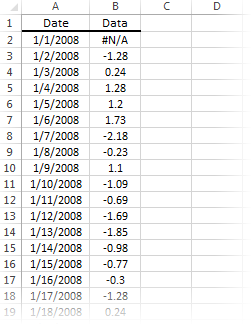Now we are ready to construct our EDF Plot First, select the empty cell in your worksheet where you wish the output table to be generated, then locate and click on the “Descriptive Statistics” icon in the NumXL tab (or toolbar). Then, select the “Empirical Distribution Function” item from the drop-down menu.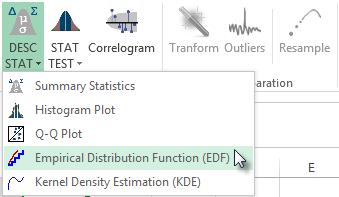The EDF Wizard pops up.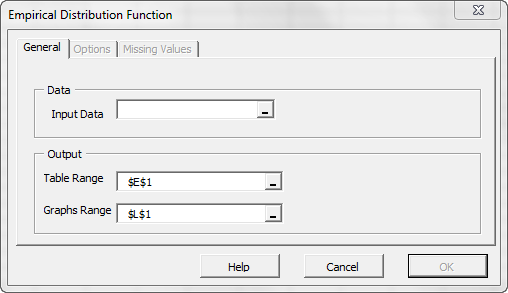Select the cells-range for the values of the input variable.

Notes:

1. The cell range includes (optional) the heading (“Label”) cell, which would be used in the output tables where it references those variables.
2. By default, the output table cells range is set to the currently selected cell in your worksheet.
3. By default, the output graph cells range is set to the 7 cells right of the currently selected cell in your worksheet.

Finally, once we select the input data (X) cells range, the “Options” and “Missing Values” tabs become available (enabled).

Next, select the “Options” tab.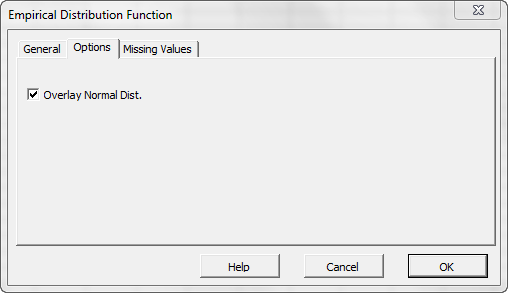Initially, the tab is set to the following values:

• “Overlay Normal distribution” is checked. This option in effect instructs the wizard to generate a second curve for the Gaussian distribution for comparison purposes. Leave this option checked.

Now, click on the “Missing Values” tab.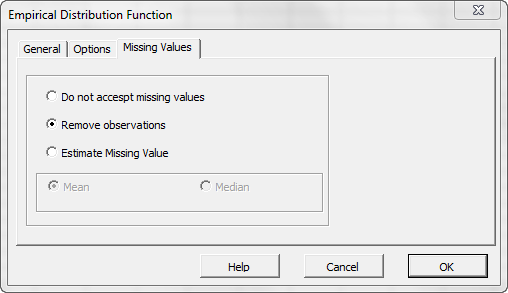In this tab, you can select an approach to handle missing values in the data set (X’s). By default, any observation with a missing value would be excluded from the analysis.

This treatment is a good approach for our analysis, so let’s leave it unchanged.

## Output

Now, click “OK” to generate the output tables.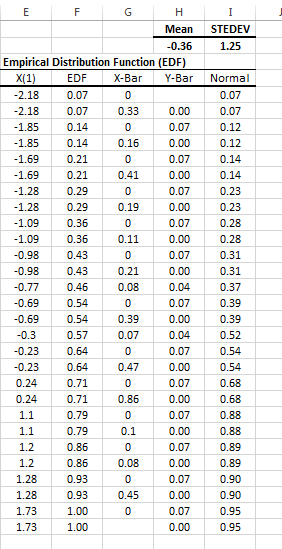Notes:

1. The values of all observations are sorted in ascending order and placed in column E.
2. The X-Bar and Y-Bar columns carry no special statistical meaning; they are merely computed to assist us in generating a step-wise type of graph in Excel.
3. Finally, the equivalent cumulative density function (CDF) of the normal distribution is computed in column I.

The generated plot of the EDF is shown below: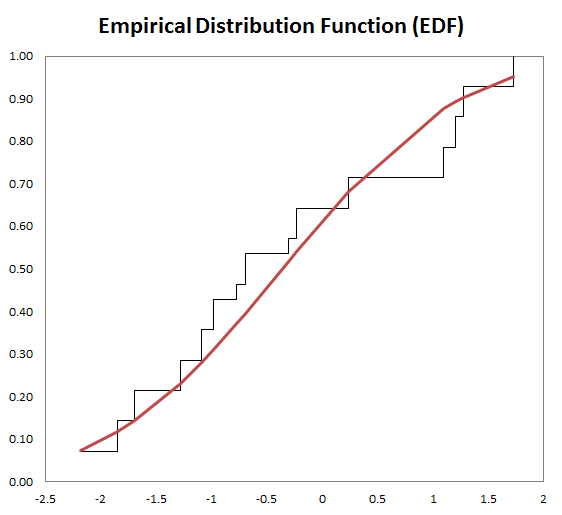## Conclusion

In this tutorial, we demonstrated the process to generate an empirical distribution function in Excel using NumXL’s add-in functions.

Where do we go from here?

To obtain the probability density function (PDF), one needs to take the derivative of the CDF, but the EDF is a step function and differentiation is a noise-amplifying operation. As a result, the consequent PDF is very jagged and needs considerable smoothing for many areas of application.

In our next entry, we will look at the kernel density estimation method to obtain the probability density function of the underlying random process.